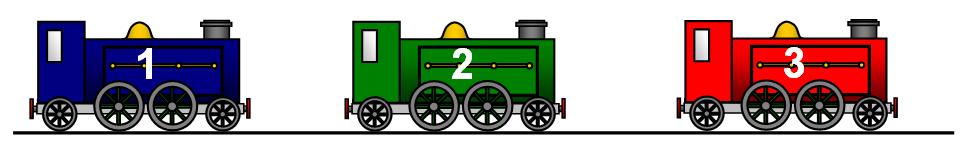# FORCES AND ENERGY

Suppose that you have three clockwork trains, all of the same mass but with their springs all wound up differently. The more the spring is wound up the more energy is stored in it.Train 1 has 1 unit of stored energy, train 2 has 2 units of stored energy and train 3 had 3 units of stored energy. All three trains are now allowed to run along a straight, level track until they stop - they will then have used up all their stored energy.

We find that the first train with 1 unit of stored energy goes 30 m before it stops.

The train stops because it has used up its stored energy in doing work against the FRICTIONAL FORCE that is trying to slow it down. This energy is converted to heat.

How far will the other trains go before they stop is the force of friction on all of them is the same.

If the trains were twice as heavy the force of friction would be twice as big and if they were three times as heavy it would be three times as big.

The energy used up by the train controls the distance travelled and this distance is also affected by the force that the train has to overcome. The train has used up some stored energy and has done work against a frictional force.

QUANTITY OF ENERGY = FORCE x DISTANCE TRAVELLED

In other words a certain amount of energy has been changed from one form to another and a certain amount of work has been done.

FORCE is measure in NEWTONS and DISTANCE in METRES.
ENERGY is measured in units called JOULES (J).
So:
JOULES = NEWTONS x METRES

One joule of energy is changed from one form to another when a force of one newton moves one metre.

Here are a few examples:

1 joule = 1 newton x 1 metre
2 joules = 2 newtons x 1 metre OR 1 newton x 2 metres
4 joules = 4 newtons x 1 metre OR 2 newtons x 2 metres etc.
8 joules = 8 newtons x 1 metre OR 4 newtons x 2 metres etc.

and so on!

Use the WORD version which contains a work sheet to help you understand this.

## LIFTING WEIGHTS

The Earth pulls on every object at its surface with a force that we call the weight of the object.
The force of the Earth on every kilogram at its surface is about 10 Newtons.
We usually write the strength of the Earth's field as 10 N/kg

When you lift up a 1 kg mass a distance of 1m you change 10 J of chemical energy in your body to 10 J of potential energy (ignoring heat for the moment).

© Keith Gibbs 2013